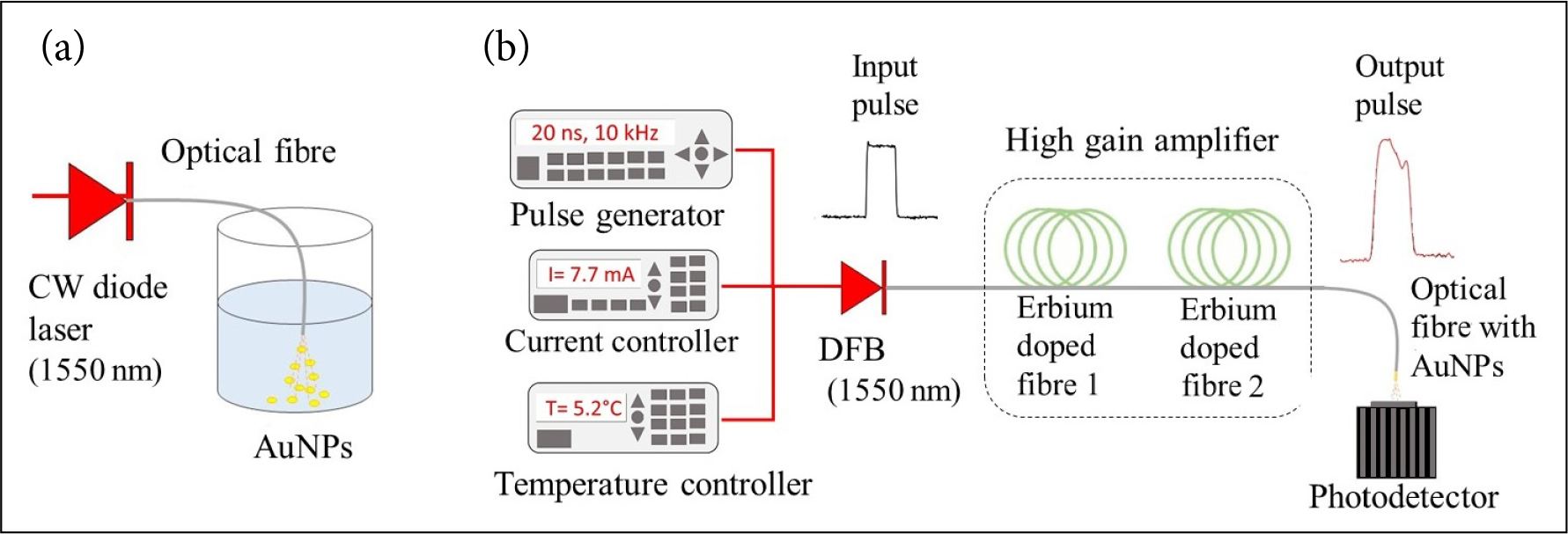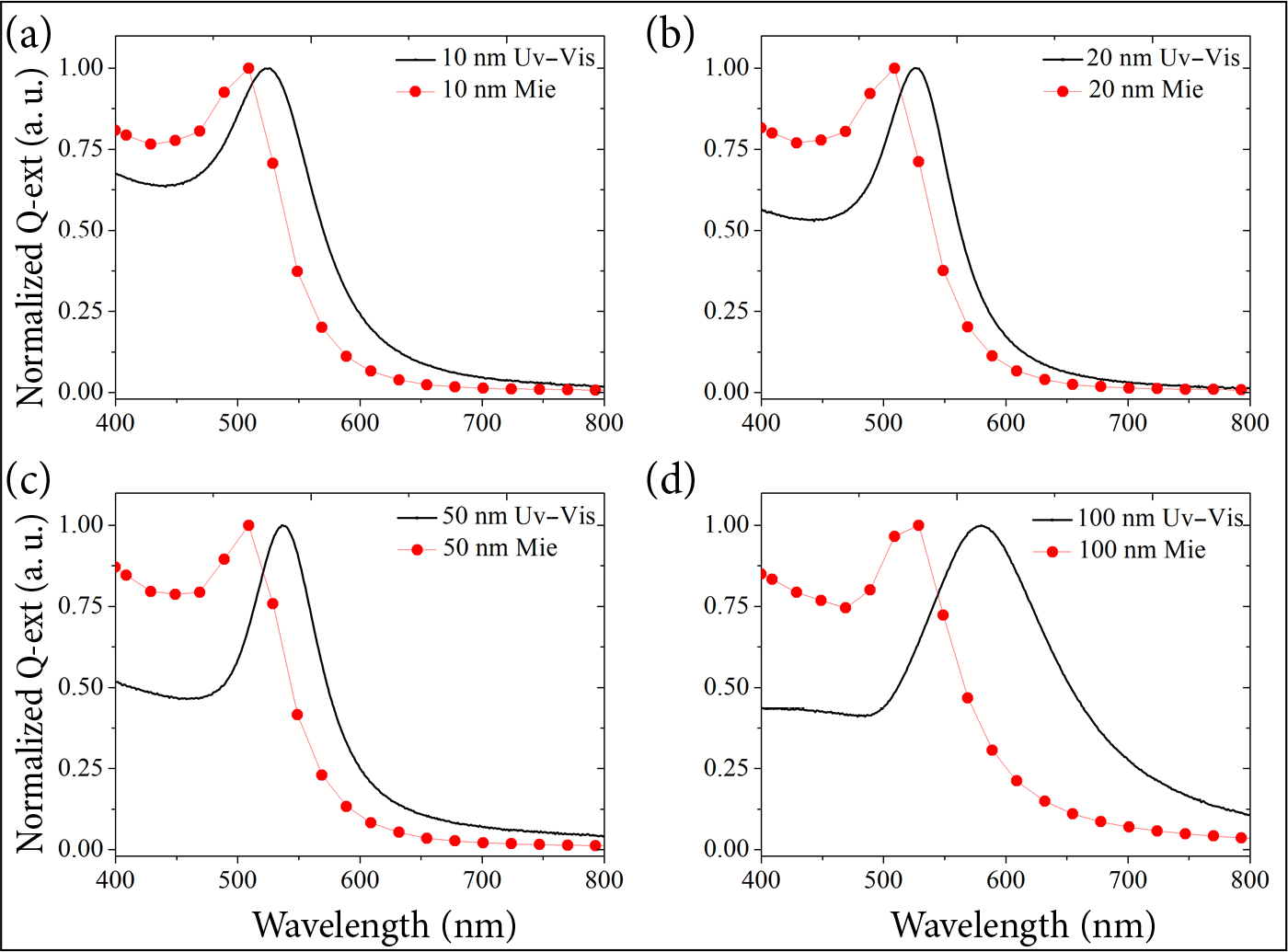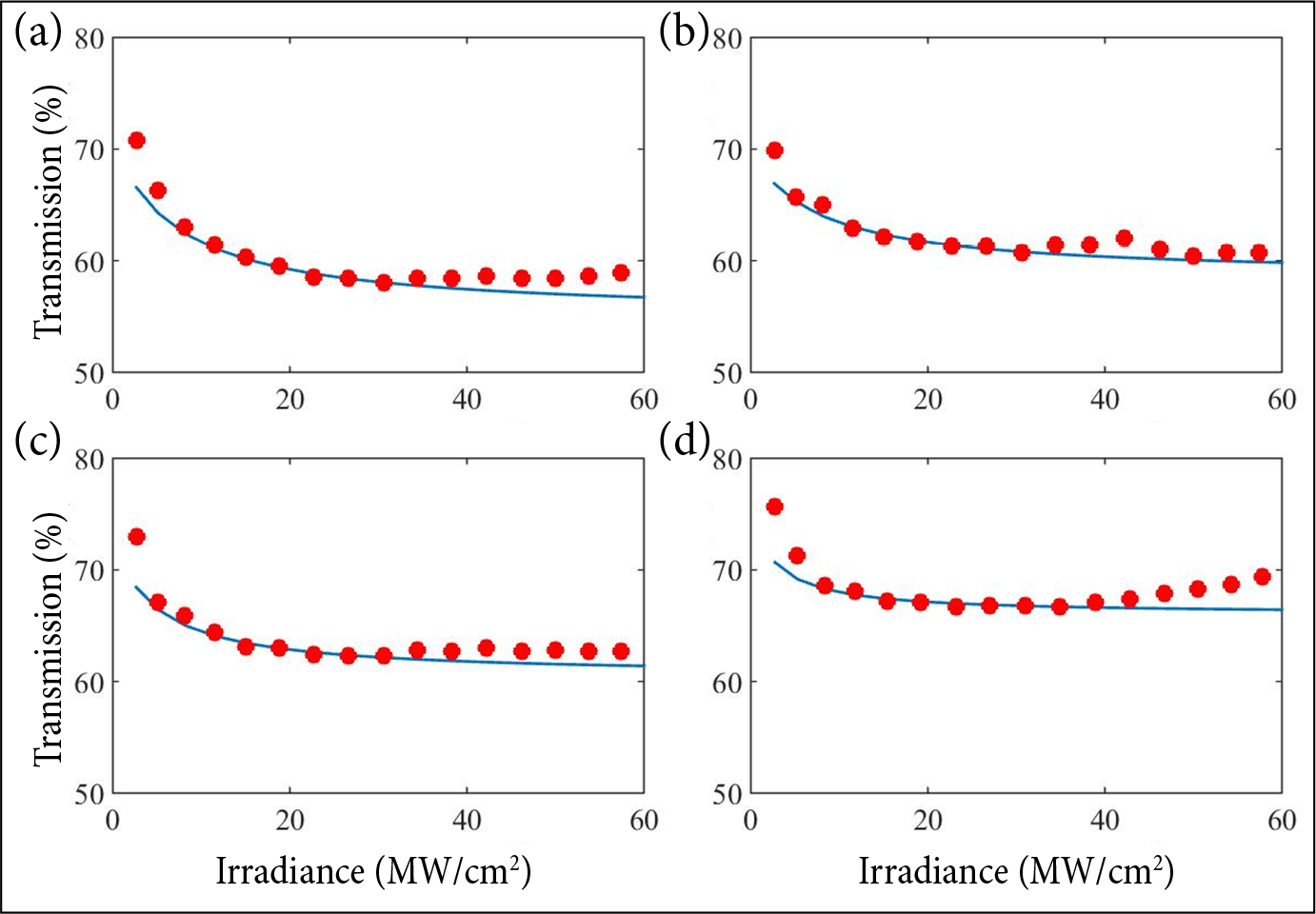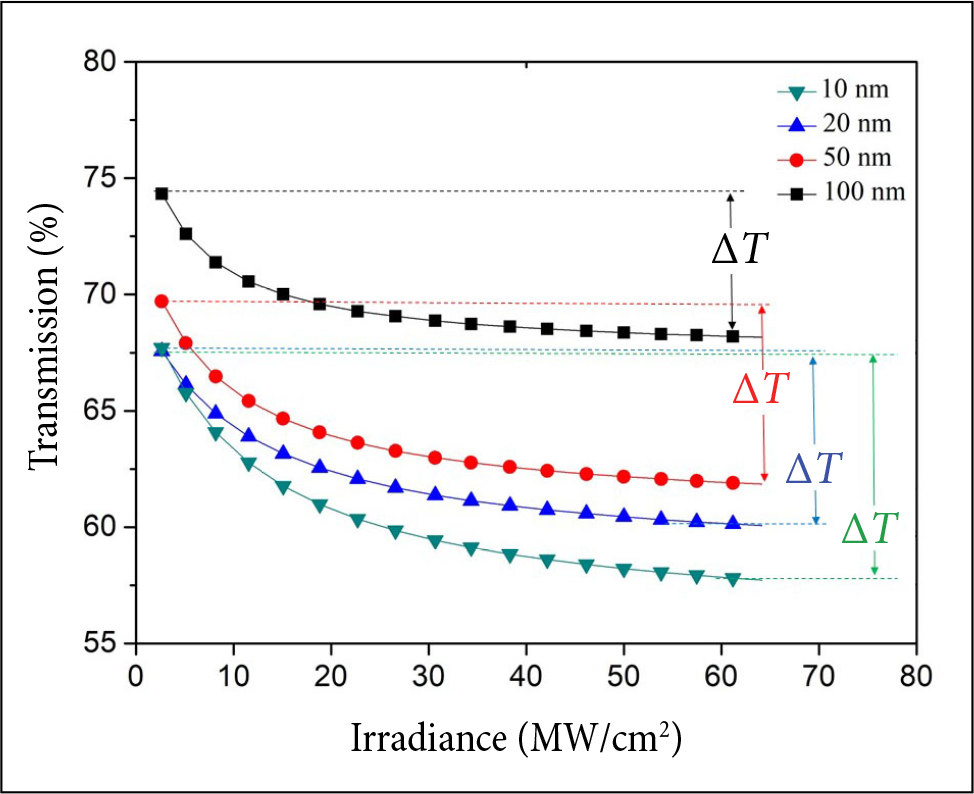﻿ MEASUREMENT OF TWO-PHOTON ABSORPTION BY GOLD NANOPARTICLES OF DIFFERENT SIZES PHOTODEPOSITED ONTO THE CORE OF AN OPTICAL FIBRE

# MEASUREMENT OF TWO-PHOTON ABSORPTION BY GOLD NANOPARTICLES OF DIFFERENT SIZES PHOTODEPOSITED ONTO THE CORE OF AN OPTICAL FIBRE

J.M. Cuvas-Limón a, J.G. Ortega-Mendoza b, J.P. Padilla-Martínez c, and P. Zaca-Morán c

a Facultad de Ciencias de la Electrónica, Benemérita Universidad Autónoma de Puebla, CP 72050, Puebla, México

b División de Posgrado, Universidad Politécnica de Tulancingo, CP 43629, Hidalgo, México

c Instituto de Ciencias, Benemérita Universidad Autónoma de Puebla, CP 72050, Puebla, México

Received 8 May 2020; revised 28 November 2020; accepted 1 December 2020

In this work, the study of two-photon absorption by gold nanoparticles of different diameters photodeposited onto the core of a single-mode optical fibre is presented. The photodeposition of nanoparticles with diameters of 10, 20, 50 and 100 nm was achieved using a continuous wave laser at a wavelength of 1550 nm and a power of 50 mW. Nonlinear optical characterization was carried out by using the P-scan technique of a high gain erbium doped fibre amplifier with pulses of 20 ns at a frequency of 10 kHz, that provides a maximum intensity of approximately 60 MW/cm2. The results show that for gold nanoparticles greater than 20 nm photodeposited onto the fibre, in both cases, the nonlinear coeﬃcient as well as the third-order susceptibility increase as the diameter of the nanoparticles increases, describing a typical behaviour of the two-photon absorption. The obtained results can be used for the design of filters and optical limiters in the communications area.

Keywords: nonlinearity, optical fibres, gold nanoparticles, photodeposition

PACS: 42.65.Yj, 42.81.-i, 81.07.-b

## 1. Introduction

The study of nanostructured materials has attracted attention in several areas of science because they have different physicochemical properties in comparison with the  bulk materials. This kind of materials is widely investigated for applications in optical storage, optical computing, harmonic generation, power limiting, rectifying devices and optical switching, therefore a high nonlinear response is a prerequisite [1-3]. Studies of the optical properties of nanomaterials, through interaction with high radiation intensities, have allowed one to know the dependence with its refractive index, giving rise to the phenomenon known as two-photon absorption (TPA) or saturable absorption (SA) [4, 5].

Currently TPA has been studied in new materials such as polymers , semiconductor materials , crystals , nanohybrid materials  and thin films  , among others, which have been used in applications as optical limiters, light sensors, tunable emitters, etc. [11-13]. Reported studies on nonlinear effects have shown that gold na-noparticles (AuNPs) can behave as a TPA material, this response depends on their morphology  [14-16], the medium in which they are embedded , the volume fraction of AuNPs in solutions  and their proportion in a solution .

In 2015, Lysenko and co-workers presented the study of the dependence of third-order susceptibility on the thickness of wafer containing layers of deposited gold . Their studies reported that the  third-order susceptibility obtained depends on the thickness of the material and the susceptibility improves when the  material is thinner. On the other hand, Maurya and co-workers published the study of TPA dependence of silver nanoparti-cles (AgNPs) and their concentration in a solution. The results show that the TPA predominates over the SA when the size of AgNPs is smaller, in addition to the SA being dominant over the TPA when the concentration is higher .

The present work gives a comparative study of the TPA at 1550 nm of AuNPs with different diameters photodeposited onto the core of a single-mode optical fibre. The nanoparticles were placed under the  influence of an infrared radiation source and their nonlinear effects were studied using a  high gain amplifier.

## 2. Experiment

Gold nanospheres were photodeposited onto the  core of an optical fibre using the  technique previously reported by a  continuous wave laser source at 1550 nm and 50 mW as is shown in Fig. 1(a) [21, 22]. AuNPs with diameters of 10, 20, 50 and 100 nm were acquired from Sigma-Aldrich company (741957, 741965, 742007 and 742031) and stabilized in a citrate buffer. The preparation of the optical fibre consisted of removing the plastic coating from the  end of the  fibre, cleaning it and making a cut on the fibre in the region where the nanospheres would be photodeposited. The fi-bre optic end was immersed into the solution with AuNPs, monitoring the  photodeposition process until the transmission decreased to 3 dB.

Figure  1(b) shows the  experimental setup to carry out the nonlinear characterization of the fi-bre with AuNPs by P-scan technique. This setup is a high gain erbium doped fibre amplifier with two amplification stages that has been reported in . In this arrangement, a  pulse is emitted by a  distributed feedback laser (DFB) that is controlled in current at 7.7 mA and stabilized in temperature at 5.2°C to emit at 1550 nm. The square pulse of 20 ns is programmed at a  frequency of 10  kHz. Under these conditions, it is possible to obtain an average power of ~7  mW that corresponds to an irradiance of ~60 MW/cm2, considering a single-mode optical fibre of 8 μm core diameter. The preparation for each fibre with AuNPs consisted of splicing the opposite end of each sample in the end of the high gain amplifier; to carry out this process, it was necessary to remove the plastic coating from the opposite end of each fibre, clean it and make a cut in the splice area to then perform the fusion using a fusion splicer. Finally, the transmission behaviours for each sample were obtained by varying the input power.

## 3. Theoretical calculations

The theoretical study was carried out using the Beer-Lambert law [21, 22], that relates the attenuation of the intensity of radiation with the properties of the material by means of the transmission. In this work, the relationship between transmittance and absorbance is approximated by the expression

$T=\mathrm{exp}\left(-\left[{\alpha }_{0}+\beta \text{I}\right]L\right),\text{ }\text{ }\text{ }\text{ }\text{ }\text{ }\text{ }\text{ }\text{ }\text{ }\text{ }\text{ }\text{ }\text{ }\text{ }\text{ }\text{ }\left(1\right)$Fig. 1. Experimental setup of (a) the photodeposition technique of AuNPs onto the core of an optical fibre and (b) a high gain erbium doped fibre amplifier to carry out the nonlinear characterization.

where L is the thickness of the sample, I is the intensity, α0 and β are the  linear and nonlinear absorption coeﬃcients, respectively. Considering a saturation model, a hyperbolic approach is used to estimate the value of the absorption coeﬃcient β,

$\beta \left(I\right)=\frac{\beta }{1+I/{I}_{\text{sat}}},\text{ }\text{ }\text{ }\text{ }\text{ }\text{ }\text{ }\text{ }\text{ }\text{ }\left(2\right)$

where Isat is the saturation intensity that is defined as the intensity when the transmission has reached 50% of the modulation. Combining the two equations we obtain the transmittance expression

$T=\mathrm{exp}\left[-\left({\alpha }_{0}+\frac{\beta }{1+I/{I}_{\text{sat}}}I\right)L\right].\text{ }\text{ }\text{ }\text{ }\text{ }\text{ }\text{ }\text{ }\text{ }\text{ }\left(3\right)$

We can use Eq. (3) to fit the experimental data of P-scan trace, and β can be treated as an adjustable parameter (Table 1). From the value of β, we can calculate the imaginary part of the third-order susceptibility by the following expression in the international system (SI) units,

$\text{Im}\left({\chi }^{\left(3\right)}\right)=\frac{\lambda {\epsilon }_{0}{n}_{0}^{2}c\beta }{4\pi },\text{ }\text{ }\text{ }\text{ }\text{ }\text{ }\text{ }\left(4\right)$

where λ is the wavelength, ε0 is the permittivity in free space, c is the speed of light in vacuum, and n0 is the refractive index of AuNPs . We can convert the units of Im(χ(3)) in (esu) using the conversion formula 1 (esu) = 1.398 × 10-8 m2/V2.

The study of the extinction coeﬃcients for different diameters of the  AuNPs was carried out theoretically by means of Mie analysis and experimentally by means of UV-Vis spectroscopy, using a  Thermo Scientific Evolution 600 spectrometer with a resolution of 1 nm and in the total transmittance mode. In order to analyse the samples, the  measurement region was from 400 to 800 nm:

${C}_{\text{ext}}=\frac{2\pi {r}^{2}}{{x}^{2}}\sum _{l=1}^{\infty }\left(2l+1\right)\mathrm{Re}\left({a}_{l}+{b}_{l}\right),\text{ }\text{ }\text{ }\text{ }\text{ }\text{ }\text{ }\text{ }\left(5\right)$
${C}_{\text{sca}}=\frac{2\pi {r}^{2}}{{x}^{2}}\sum _{l=1}^{\infty }\left(2l+1\right)\left({\left|{a}_{l}\right|}^{2}+{\left|{b}_{l}\right|}^{2}\right),\text{ }\text{ }\text{ }\text{ }\text{ }\text{ }\text{ }\left(6\right)$
${C}_{\text{abs}}={C}_{\text{ext}}-{C}_{\text{sca}}.\text{ }\text{ }\text{ }\text{ }\text{ }\text{ }\text{ }\text{ }\text{ }\text{ }\left(7\right)$

Here l denotes the multipolar order of the plasmons of excited particles, x = 2πr/λ represents a size parameter proportional to the ratio of the radius of the sphere r to the wavelength λ for a wavelength of 1550 nm and the spheres with radii of 10, 20, 50 and 100 nm. The coeﬃcients of Mie are

${a}_{l}=\frac{m{\psi }_{l}\left(mx\right){\psi }_{l}^{\prime }\left(x\right)-{\psi }_{l}\left(x\right){\psi }_{l}^{\prime }\left(mx\right)}{m{\psi }_{l}\left(mx\right){\xi }_{l}^{\prime }\left(x\right)-{\xi }_{l}\left(x\right){\psi }_{l}^{\prime }\left(mx\right)},\text{ }\text{ }\text{ }\text{ }\text{ }\text{ }\text{ }\text{ }\text{ }\left(8\right)$
${b}_{i}=\frac{{\psi }_{l}\left(mx\right){\psi }_{l}^{\prime }\left(x\right)-m{\psi }_{l}\left(x\right){\psi }_{l}^{\prime }\left(mx\right)}{{\psi }_{l}\left(mx\right){\xi }_{l}^{\prime }\left(x\right)-m{\xi }_{1}\left(x\right){\psi }_{l}^{\prime }\left(mx\right)},\text{ }\text{ }\text{ }\text{ }\text{ }\text{ }\text{ }\text{ }\left(9\right)$

expressed by the functions of Ricatti-Bessel ψl and ξl, they contain the  dependence of specific complex dielectric functions of the  material through m2 = εmetalmedium.

## 4. Results and discussion

The theoretical and experimental results for na-noparticles obtained by means of the UV-Vis and Mie analysis are shown in Fig. 2. Figures 2(a-d) show that the extinction coeﬃcients are affected by their diameters. The theoretical results by Mie analysis show a  shift of the  wavelength towards the  UV region when the  diameter of the  nano-particle decreases. These results agree with the experimental data that show an absorption peak at longer wavelengths for nanoparticles with large diameters. This absorption peak also shows a shift towards the UV region for smaller nanoparticles. This behaviour is a well-known phenomenon that is attributed to the absorption by AuNPs, extinction coeﬃcient and consequently to the  effect of surface resonance plasmon (SPR)  . Studies have shown that the  changes in the  value of the SPR peak are due to the morphology, geometric dimensions and dielectric constants of a material [15, 24]. Additionally, it has been reported that in metals the SPR peak is sensitive to the size and amount of fraction of the particles that cause a change in the wavelength .

The nonlinear transmission as a function of ir-radiance is shown in Fig. 3. In this plot, the dotted lines indicate the  transmittance obtained experimentally, and the solid lines indicate the transmittance obtained using the  Beer-Lambert law. According to the  results shown, it can be seen that when an optical fibre with AuNPs is irradiated by the 20 ns pulses of 1550 nm laser source, the transmittance decreases linearly in the  region of low irradiance; on the  other hand, the  results show a  decrease in transmission as intensity increases, showing that the AuNPs of 10 nm have the lowest level of transmission and the AuNPs 100 nm have the highest level.Fig. 2. Extinction coeﬃcients as a function of the wavelength of AuNPs with diameters of (a) 10 nm, (b) 20 nm, (c) 50 nm and (d) 100 nm.

Figure 4 depicts the  nonlinear transmission behaviours of each sample as a  function of irra-diance using the  Beer-Lambert law. The  results show that the decrease in the transmission at low intensities for each nanoparticle size has different saturation intensities. The nanoparticles of 10, 20, 50 and 100 nm show saturation intensities of 8.2, 8.1, 5.1 and 2.7 W/cm2, respectively, implying that small AuNPs have the ability to gradually absorb more intense amounts of radiation before saturating. This optical limiting mechanism is attributed to the combination of laser induced AuNPs dispersion and the nonlinear absorption. In addition, it can be observed that the saturation intensity and the  modulation depth (ΔT) increase for smaller AuNPs diameters, these results are due to the Isat greater magnitude. Consequently the  linear absorption coeﬃcient α0 increases, respectively, as shown in Table 1. This dependence of the depth of modulation on the diameter of the sphere, that is inversely proportional, indicates that it can be used for the  design of lasers, optical limiters and light sensors, among others. All the results show a typical behaviour of the TPA curve .Fig. 3. Transmission as a function of the irradiance of the AuNPs of (a) 10 nm, (b) 20 nm, (c) 50 nm and (d) 100 nm.Fig. 4. Transmission adjustment based on the irradiance of AuNPs.

In addition, the  results show that for AuNPs greater than 20 nm the nonlinear coeﬃcient β and third-order susceptibility increase as the diameter of the  nanoparticles increases, that indicates that the TPA improves since its capacity of optical limi tation increases . Optical limiting occurs when the  optical transmission of a  material decreases with increasing laser fluence, a property that is desirable for protection of sensors and human eyes from intense laser radiation.

On the  other hand, it is important to mention that the advantage of using the technique of photodeposition of nanostructured materials on the nucleus of optical fibres is the minimal use of nanostructured materials, in contrast to the devices made on films, crystals or solutions. This technique allows one, as it has been demonstrated, to have a better control of the nonlinear parameters of the  materials placed on the  fibres, that makes it ideal for the  development of photonic devices. Clearly, the AuNPs endowed the fibres with a limiting power due to the optical limiting mechanisms for AuNPs that are quite different from those of carbon nanotubes.

## 5. Conclusions

The AuNPs photodeposited onto the core of a single-mode optical fibre exhibit nonlinear properties depending on the  dimension of their diameters. Maintaining a constant transmission in each fibre sample with AuNPs, the nonlinear optical charac-terization was performed for diameters between 10 and 100 nm. The results obtained in our experiments showed a typical behaviour of two-photon absorption, achieving saturation when the intensity increased. Additionally, it was observed that the  modulation depth turned out to be greater when the  diameter of the  gold nanoparticle was smaller. To our knowledge, the study of the nonlinear dependence of this type of nanomaterials photodeposited on an optical fibre has not been reported, which can be used for the implementation of photonic devices based on nanostructured materials and optical fibres.

Table 1. Absorption coeﬃcients and third-order susceptibility of the different diameters of AuNPs.
Diameter (nm) α0 (1/m) ΔT (%) β (m/W) × 10-6 χ(3) (esu) × 10-7
10 3.055 11.48 3.084 1.9810
20 3.588 10.16 2.152 1.3824
50 3.153 10.14 3.659 2.3500
100 2.794 7.97 4.956 3.1832

## Acknowledgements

This research was supported by Fondo Institucional del Conacyt (FOINS) under Grant No. 2319.

## References

 Y. Golian and D. Dorranian, Effect of thickness on the optical nonlinearity of gold colloidal na-noparticles prepared by laser ablation, Opt. Quant. Electron. 46, 809-819 (2014), https://doi.org/10.1007/s11082-013-9792-z

 C. Li, Y. Qi, X. Hao, X. Peng, and D. Li, Gold na-norods-silicone hybrid material films and their optical limiting property, Appl. Phys. A 121, 11-15 (2015), https://doi.org/10.1007/s00339-015-9401-z

 N.  Vijayan, G.  Bhagavannarayana, S.K.  Halder, S. Verma, J. Philip, R. Philip, and B. Rathi, X-ray topography, photopyroelectric and two-photon absorption studies on solution grown benzimida-zole single crystal, App. Phys. A 110, 55-58 (2013), https://doi.org/10.1007/s00339-012-7448-7

 O.M. Bankole and T. Nyokong, Mercatopyridine-substituted indium, zinc, and metal-free phtha-locyanines: nonlinear optical studies in solution and on polymer matrices, J. Coord. Chem. 68, 3727-3740 (2015), https://doi.org/10.1080/00958972.2015.1077237

 K.V. Anand, G. Vinitha, M.K. Chinnu, R. Mohan, and R.  Jayavel, Enhanced third-order nonlinear optical properties of high purity ZnS na-noparticles, J. Nonlinear Opt. Phys. Mater. 24(02), 1-11 (2015), https://doi.org/10.1142/S0218863515500162

 M.S. Sunitha, K.A. Vishnumurthy, and A.V. Adhi-kari, Synthesis and two-photon absorption property of new π-conjugated donor-acceptor polymers carrying different heteroaromatics, J. Chem. Sci. 125, 29-40 (2013), https://doi.org/10.1007/s12039-013-0366-1

 P.P.  Kiran, S.V.  Rao, M.  Ferrari, B.M.  Krishna, H. Sekhar, S. Alee, and D.N. Rao, Enhanced optical limiting performance through nonlinear scattering in nanoparticles of CdS, co-doped Ag-Cu, and BSO, Nonlinear Opt. Quantum Opt. 40, 223-234 (2010).

 V.I.  Lukanin, D.S.  Chunaev, and A.Y.  Karasik, Two-photon absorption of high-power pico-second pulses in PbWO4, ZnWO4, PbMoO4, and CaMoO4 crystals, J. Exp. Theor. Phys. 113(3), 412-421 (2011), https://doi.org/10.1134/S1063776111070077

 Q. Zhang, X. Tian, H. Zhou, J. Wu, and Y. Tian, Lighting the way to see inside two-photon absorption materials: structure-property relationship and biological imaging, Materials 10(3), 1-37 (2017), https://doi.org/10.3390/ma10030223

 R.A.  Ganeev, A.I.  Ryasnyanskii, M.K.  Kodirov, S.R.  Kamalov, and T.  Usmanov, Optical limiting in fullerenes, colloidal metal solutions, and semiconductors in the  field of pico- and nanosecond pulses of an Nd:YAG laser, Opt. Spectrosc. 93(5), 789-796 (2002), https://doi.org/10.1134/1.1524003

 S.  Hirata, K.  Totani, T.  Yamashita, C.  Aadachi, and M. Vacha, Large reverse saturable absorption under weak continuous incoherent light, Nat. Mat. 13, 938-946 (2014), https://doi.org/10.1038/nmat4081

 R.A. Silva and M.O. Orlandi, Influence of synthesis route on the radiation sensing properties of ZnO nanostructures, J. Nanomater. 2016, 1-9 (2016), https://doi.org/10.1155/2016/4054058

 L.  Irimpan, V.P.N.  Nampoori, and P.  Radha-krishnan, Spectral and nonlinear optical characteristics of ZnO nanocomposites, Sci. Adv. Mater. 2(2), 117-137 (2010), https://doi.org/10.1166

 S. Kumar, M. Anija, and A.K. Sood, Tuning ultra-fast photoresponse of gold nanorods, Plasmonics 8, 1477-1483 (2013), https://doi.org/10.1007/s11468-013-9561-7

 T. Cesca, P. Calvelli, G. Battaglin, P. Mazzoldi, and G. Mattei, Nonlinear optical response of gold-silver nanoplanets, Radiat. Eff. Defect. S. 167(7), 520-526 (2012), https://doi.org/10.1080/10420150.2012.680458

 H.I. Elim, J. Yang, and J.Y. Lee, Observation of saturable and reverse-saturable absorption at longitudinal surface plasmon resonance in gold nanorods, Appl. Phys. Lett. 88(8), 083107 (2006), https://doi.org/10.1063/1.2177366

 Y.  Yang and M.  Nogami, Controlled surface-plasmon coupling in SiO2-coated gold nano-chains for tunable nonlinear optical properties, Appl. Phys. Lett. 88, 081110 (2006), https://doi.org/10.1063/1.2172022

 J. Zhu, S.W. Bai, J.W. Zhao, and J.J. Li, Tunable optical limiting of gold nanorod thin films, Appl. Phys. A 97, 431-436 (2009), https://doi.org/10.1007/s00339-009-5232-0

 O. Lysenko, M. Bache, and A. Lavrinenko, Third-order susceptibility of gold for ultrathin layers, Opt. Lett. 41(2), 317-320 (2016), https://doi.org/10.1364/OL.41.000317

 S.K.  Maurya, A.  Rout, R.A.  Ganeev, and C.  Guo, Effect of size on the  saturable absorption and reverse saturable absorption in silver nanoparticle and ultrafast dynamics at 400 nm, J.  Nanomater. 2019, 1-12 (2019), https://doi.org/10.1155/2019/9686913

 P.  Zaca-Morán, R.  Ramos-Garcia, J.G.  Ortega-Mendoza, F.  Chávez, G.F.  Pérez-Sánchez, and C.  Felipe, Saturable and two-photon absorption in zinc nanoparticles photodeposited onto the core of an optical fiber, Opt. Express 23(14), 18721-18729 (2015), https://doi.org/10.1364/OE.23.018721

 L.C. Gómez-Pavón, G.J. Lozano-Perera, A. Luis-Ramos, J.M.  Muñoz-Pacheco, J.P.  Padilla-Mar-tí nez, and P. Zaca-Morán, Influence on the sat-urable absorption of the  induced losses by photodeposition of zinc nanoparticles in an optical fiber, Opt. Express 26(2), 1556-1563 (2018), https://doi.org/10.1364/OE.26.001556

 P.  Zaca-Morán, E.  Kuzin, J.  Torres-Turiján, J.G.  Ortega-Mendoza, F.  Chávez, G.F.  Pérez-Sánchez, and L.C.  Gómez-Pavón, High gain pulsed erbium-doped fiber amplifier for the nonlinear characterization of SWCNTs photodepos-ited on optical fibers, Opt. Laser Technol. 52, 15-20 (2013), https://doi.org/10.1016/j.opt-lastec.2013.04.004

 A.  Alesenkov, J.  Pilipavičius, A.  Beganskienė, R. Sirutkaitis, and V. Sirutkaitis, Nonlinear properties of silver nanoparticles explored by a fem-tosecond Z-scan technique, Lith. J. Phys. 55(2), 100-109 (2015), https://doi.org/10.3952/physics.v55i2.3100

 S. Mohapatra, Y.K. Mishra, A.M. Warrier, R. Phil-ip, S.  Sahoo, A.K.  Arora, and D.K.  Avasthi, Plasmonic, low frequency Raman, and nonlinear optical-limiting studies in copper-silica nano-composites, Plasmonics 7, 25-31 (2012), https://doi.org/10.1007/s11468-011-9271-y

### FOTODEPOZICIJOS BŪDU ANT OPTINIO SVIESOLAIDZIO SERDIES NUSODINTŲ AUKSO DALELIŲ DVIFOTONĖS ABSORBCIJOS MATAVIMAI

J.M. Cuvas-Limón a, J.G. Ortega-Mendoza b, J.P. Padilla-Martínez c, P. Zaca-Morán c

a Autonominio Pueblos universiteto Elektronikos fakultetas, Puebla, Meksika

b Tulancingo politechnikos universitetas, Hidalgas, Meksika

c Autonominio Pueblos universiteto Mokslo institutas, Puebla, Meksika